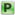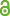Hauptmenü
• Autor
• Ejsmont, Wiktor
• Lehner, Franz
• TitelSample Variance in Free Probability
• Datei
• Persistent Identifier
• Erschienen inarXiv.org
• Erscheinungsjahr2016
• LicenceCC-BY
• Zugriffsrechte• AbstractLet $X_1, X_2,\dots, X_n$ denote i.i.d.~centered standard normal random variables, then the law of the sample variance $Q_n=\sum_{i=1}^n(X_i-\bar{X})^2$ is the $\chi^2$-distribution with $n-1$ degrees of freedom. It is an open problem in classical probability to characterize all distributions with this property and in particular, whether it characterizes the normal law. In this paper we present a solution of the free analog of this question and show that the only distributions, whose free sample variance is distributed according to a free $\chi^2$-distribution, are the semicircle law and more generally so-called \emph{odd} laws, by which we mean laws with vanishing higher order even cumulants. In the way of proof we derive an explicit formula for the free cumulants of $Q_n$ which shows that indeed the odd cumulants do not contribute and which exhibits an interesting connection to the concept of $R$-cyclicity.